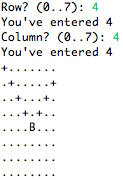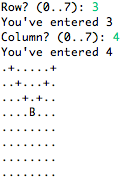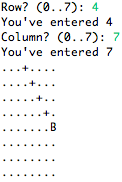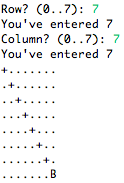## Thursday, March 14, 2013

### Chess in C (Part 6) - Potential moves of a bishop: up-right

This post is a continuation of Chess in C (Part 4). No fancy introductions or recaps. If you don't read that, you won't understand this.

Mission: write an algorithm that returns the up-right diagonal from a bishop in position (X,X).

We need to get (2,5), (1,6) and (0,7). We can modify the algorithm used to figure out the up-left diagonal as follows
1. Start at the last square and iterate through the board backwards
2. For each square, check if the upper-right square is within the board
1. If it is, check if there's a bishop or breadcrumb in the upper-right square
1. If there is, this square is a potential move. Mark this square with a breadcrumb.
3. Repeat until you reach (0,0).
Just like in the up-left algorithm, we need to iterate through the board backwards. If we iterate forwards, we won't pickup all the potential moves. Here's an illustration: start at square (0,0). Ask yourself, "Is there a bishop or breadcrumb in the lower-left diagonal square?" Progress to the next square. Ask yourself the same question. At position (0,7), your answer will still be no, even though (0,7) is a potential move!You call yourself a bishop?!.

This is what happens when we iterate backwards.

The code to implement this algorithm is similar to the up-left diagonal. Unfortunately, it's a little too similar!

If we reuse the code, the breadcrumbs for each of the algorithms will trip up over each other! The solution is to modify the macros and board drawing code to differentiate between the breadcrumbs of each algorithm. I've made a few more POTENTIAL_BISHOP_MOVE macros and appended _UL (upper-left diagonal), _UR (upper-right diagonal), _DL (down-left diagonal) and _DR (down-right diagonal) to them. Here's the code you'll need to begin this lesson.

Now it's time to code up this algorithm.

The code is similarly straight forward. Lines 4 and 5 contain the for loops which iterate through each element in the array backward. Line 8 prevents us from checking the row below or the cell to the left if it doesn't exist. Imagine we're in square (2,5). Because we want to know if the square is a potential up-right diagonal move of a bishop, the bishop must be in the lower-left direction. Here's an illustration.Put your finger on square (2,5). Yes, it's covered by an X. Pretend you don't know where the bishop is. If you were trying to find out of the square you're in (2,5) was up-right of a bishop, which way would you search?

The location of the bishop relative to the X is (+1,-1). We're at (2,5), so we're checking if (3,4) exists. Which it does. But what if we were at square (7,0)? Square (8,-1) definitely doesn't exist, and if we try to check that square we'll exceed the bounds of the array. Which is bad.

Line 10 checks the actual cell for a bishop or a breadcrumb. If it does, line 12 marks the square with a breadcrumb for the board drawing code to interpret. Time to test!Bishop in position (0,0). You know what we're not doing? Testing if there's a bishop in (1,-1)Bishop in position (4,4). It looks like the algorithm has been implemented correctly.Bishop in position (3,4). That looks nice!Bishop in position (4,7). We're testing an edge case. It works.Bishop in position (7,7). Another edge case to test. And it works!
Done! Here's the code.